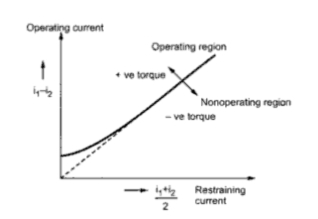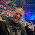Basic Percentage Differential protection Scheme for Generators

Generator Protection Part 4

Basic Percentage Differential protection Scheme for Generators
This protection scheme is also called biased differential protection scheme. The Fig. 1 shows the connection of the percentage differential relay, in such a protection scheme.Fig. 1

The fault occurs at point X and the primary currents in the circuit are I1 and I2. The C.T. secondary currents are i1 and i2. The current flowing through the operation coil of the relay is (i1 + i2)/2. This is because the operating coil is connected to the midpoint of the restraining coil.
Thus if the number of turns of the restraining coil is N then i1 flows through N/2 and i2 flows through remaining N/2. The total ampere turns are i1N/2+ i2N/2 i.e N ((i1 + i2)/2). This is as good as the flow of current (i1 + i2)/2 through the entire restraining coil.
The operation characteristics of such a biased differential relay is shown in the Fig 2. The characteristics shows that except at low currents, ratio of differential operation current to average restraining current is a fixed percentage. Hence the relay is called the percentage differential relay.Fig. 2

This basic percentage differential protection scheme forms the basis of the practically very commonly used percentage differential protection scheme for alternator stator windings. This popular scheme is known as Biased differential protection or Merz-Price protection.
Let us discuss the details of Merz-Price protection scheme for the three phase alternator stator windings in the next post.

1."The current flowing through the operation coil of the relay is (i1 + i2)/2. This is because the operating coil is connected to the midpoint of the restraining coil."

I am reading the Art & Science of Protective Relaying book right now, and I think that this portion of your entry is incorrect. From the book...

"The differential current in the operating coil is proportional to I1 - I2, and the equivalent current in the restraining coil is proportional to (I1 + I2)/2, since the operating coil is connected to the midpoint of the restraining coil."

2.Yes!
You are right Shane.

3.Yes!
You are right Shane.